# Battery Internal Resistance

• B
• neilparker62
The lead connecting to the load resistor is clipped onto the probe. So that the load only draws current when I touch the probe(s) onto the battery terminal(s). It's the low resistance load which is drawing heavy current - not the multimeter which has high impedance as you indicated.There is non-negligible series resistance in the circuit, even when R =...In summary, the student is investigating the internal resistance of an AA 1.5 V battery. They have been having difficulty measuring the load voltage accurately and have been investigating ways to improve the situation. They have found that there is non-negligible series resistance in the circuit, even when R =...

#### neilparker62

Homework Helper
TL;DR Summary
Ohm's law offset voltage
Have been busy working with a student doing a Physics prac to determine the internal resistance of an AA 1.5 V (nominal) battery. The basic formula being investigated is:

$$Vt=E_{cell}−Ir_i$$ where ##Vt## is the load (resistive only) voltage, ##E_{cell}## is the emf of the AA battery, ##I## is the circuit current and ##r_i## the internal resistance of the battery.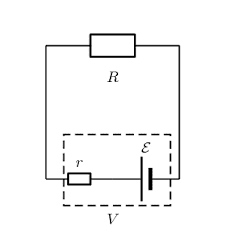Practical problems we have had is that the terminal voltage seems to fluctuate continuously when you try to measure it. This can be mitigated somewhat by increasing the load resistance but then you start finding that the internal voltage drop is close to the limit of voltmeter resolution. R≈30Ω seems to be a reasonable value. That seems to be what is used on the "battery test" setting on my multimeter since the manual indicates an approximate current draw of 50 milli amps. (1.5 / 30).

However the specific issue I would like to ask advice on here is that I am consistently finding discrepancies between load resistance and the quotient of (measured) load volts over (measured) amps. The regression gradient is more or less unity as expected but there is a distinct offset value of nearly 2 ohms which I cannot explain. In terms of the determination of ##r_i## this is a problem since there is wide divergence between ##\Delta V## determined as ##E_{cell}-V_t## and as ##E_{cell}-IR##.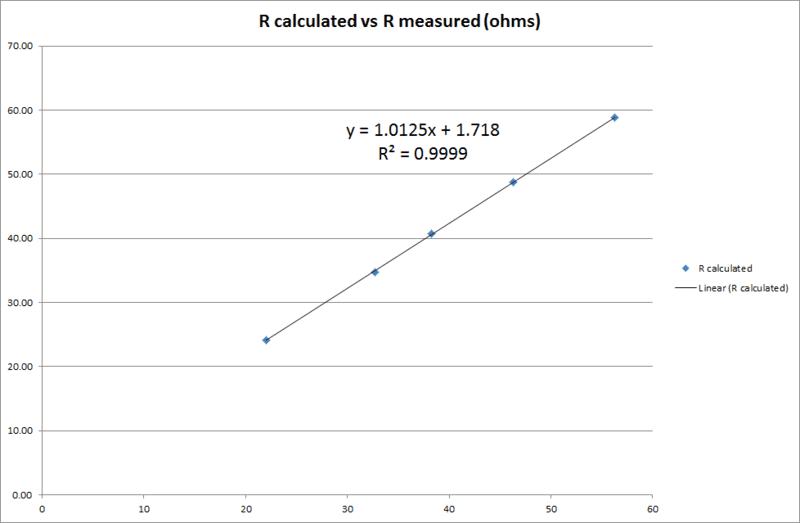Here is my regression curve (R calculated vs R measured). Am using fixed value resistors but measuring anyway.

Last edited:
•Delta2
Regarding the fluctuating terminal voltage: what does your physical setup look like? Are you just trying to hold the multimeter probes to the ends of the battery with your hands? Is this something you could fix simply by throwing in some flying leads and alligator clips or banana plugs to connect to them, so that you could keep the DMM stable on the bench top?

LastScattered1090 said:
Regarding the fluctuating terminal voltage: what does your physical setup look like? Are you just trying to hold the multimeter probes to the ends of the battery with your hands? Is this something you could fix simply by throwing in some flying leads and alligator clips or banana plugs to connect to them, so that you could keep the DMM stable on the bench top?
I am using crocodile clips and I did carefully consider bad connections as a source of voltage fluctuations. Initially we planned to use low resistance values (< 10 ohms) but then it becomes almost impossible to measure load voltage since the large current draw is literally dropping the voltage (if not emf as well) as you watch.

Yes I am touching the probes to the battery by hand since one has to 'touch and release' otherwise the battery quickly gets hammered by the large current draw.

It's hard to know what's going on with your plot without understanding how you are arriving at R_calculated. Can you give us some more details on that?

The math can be expanded to

$$V_t = E_\mathrm{cell} - Ir_i = E_\mathrm{cell} - \left(\frac{E_\mathrm{cell}}{R+ri}\right)r_i$$

$$V_t = E_\mathrm{cell}\left(1 - \frac{r_i}{R+ri}\right)$$

if that helps.

I'm surprised to hear you say that the battery gets hammered when you connect the DMM. In voltmeter mode, a DMM should have a large input impedance, so that it doesn't place a big parasitic load on the circuit. It won't draw zero current, but it should not draw much. Are you connecting the DMM across the battery terminals, in parallel with the load resistor R? Sorry to question this, I mean no offence. Just thought it couldn't hurt to sanity check the basics.

•neilparker62 and nasu
The lead connecting to the load resistor is clipped onto the probe. So that the load only draws current when I touch the probe(s) onto the battery terminal(s). It's the low resistance load which is drawing heavy current - not the multimeter which has high impedance as you indicated.

R calculated is simply ##\frac{V_t}{I}## where ##V_t## is the measured load voltage and ##I## the measured load current.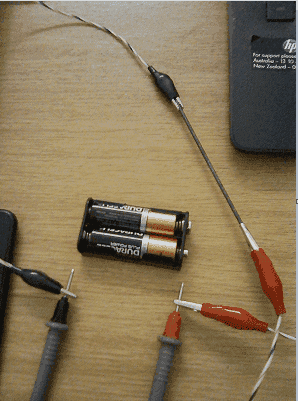•Delta2
neilparker62 said:
The basic formula being investigated is:

$$Vt=E_{cell}−Ir_i$$ where ##Vt## is the load (resistive only) voltage, ##E_cell## is the emf of the AA battery, ##I## is the circuit current and ##r_i## the internal resistance of the battery.
Shouldn't this basic formula also have a term for the lead resistance which you can determine separately?

neilparker62 said:
The lead connecting to the load resistor is clipped onto the probe. So that the load only draws current when I touch the probe(s) onto the battery terminal(s). It's the low resistance load which is drawing heavy current - not the multimeter which has high impedance as you indicated.

R calculated is simply ##\frac{V_t}{I}## where ##V_t## is the measured load voltage and ##I## the measured load current.
My best guess is non-negligible series resistance in the circuit, even when R = 0.

The drop in the load voltage is not a fluctuation. It is normal behavior of the battery. The voltage decreases with time and the decrease rate is faster for larger currents. This is what you see during some battery tests based on cyclic charge and discharge. If you want a practically constant voltage over the duration of your test you need to draw an appropriate low current.

•Delta2
The internal resistance of the battery is a variable, not a parameter.
I would be concerned that the internal battery temperature will change during high current measurements. The time response of the current measurement should be investigated. That will be determined by the diffusion of available chemicals within the cell, which will be time dependent in the short term, and history dependent in the long term.

•berkeman
^^^^^^
What Baluncore said.

neilparker62 said:
Have been busy working with a student doing a Physics prac to determine the internal resistance of an AA 1.5 V (nominal) battery.
Under what conditions? The "internal resistance" of the battery as well as the battery's inherent "source voltage" depend on a number of variables that you don't seem to mention or try to account for. What exactly is the problem statement for the lab? Is there a suggested procedure?

kuruman said:
Shouldn't this basic formula also have a term for the lead resistance which you can determine separately?
With the setup as shown above, I can first measure resistance. Since the leads (and probe leads) are part of the circuit, they should automatically be included within the measurement although (hopefully) they have negligible resistance anyway.

berkeman said:
^^^^^^
What Baluncore said.

Under what conditions? The "internal resistance" of the battery as well as the battery's inherent "source voltage" depend on a number of variables that you don't seem to mention or try to account for. What exactly is the problem statement for the lab? Is there a suggested procedure?
This is a basic school Science prac. I'm aware of more complex circuit models for typical AA cell batteries.

http://mocha-java.uccs.edu/ECE5710/ECE5710-Notes02.pdf

But we do not want to do much more than measure the voltage drop under load and divide by current to obtain an estimate of internal resistance. Therefore the aim is to try and provide experimental conditions under which internal resistance is the dominant cell parameter (*) and holds more or less constant. Internal heating of the cell due to heavy current draw is probably not ideal in that respect which is why I advised my student to use higher resistance values than advised in the prac sheet (4 ohms).

(*) I take the point that this is a variable rather than a parameter but that's why we're trying to find conditions under which it is constant.

From my own experimenting, I found that current readings generally do not fluctuate as much as load or terminal voltage readings so I thought it might be best to obtain a calculated value of load voltage (IR) rather than use the measured value. That led me to check if there was basic adherence to ohm's law (load voltage / current = resistance) with results as shown in my graph in post #1.

One can also plot load volts vs current whereby the gradient is the negative of internal resistance and the y-intercept is emf. Graph follows - it is not too bad but I hoped for a better correlation coefficient and an emf (open circuit voltage) value closer to that measured.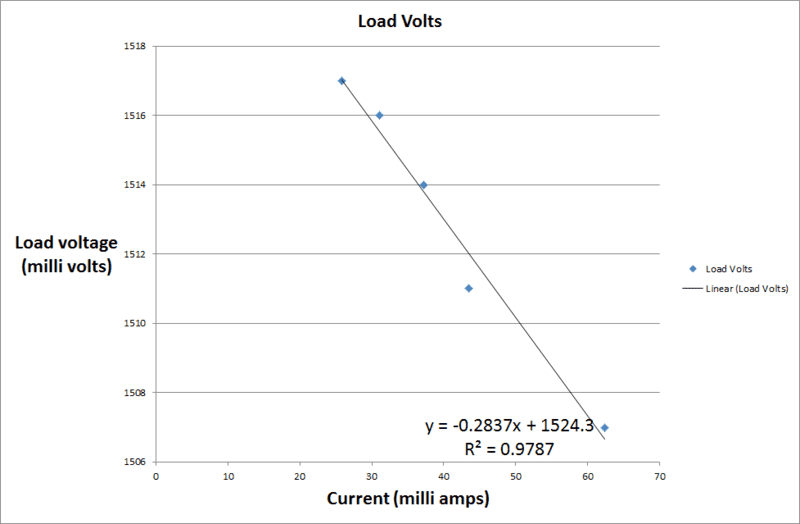nasu said:
The drop in the load voltage is not a fluctuation. It is normal behavior of the battery. The voltage decreases with time and the decrease rate is faster for larger currents. This is what you see during some battery tests based on cyclic charge and discharge. If you want a practically constant voltage over the duration of your test you need to draw an appropriate low current.
I understand that. We do expect a drop in voltage under load due to internal resistance of the cell. And it's that load voltage we are trying to measure and which is fluctuating. The cell emf itself (open circuit voltage) does not fluctuate but it does gradually decrease with amp hours usage. That's another reason to avoid high currents because then amp hours capacity drops rapidly and emf with it.

The voltage under load for a given load decreases as well. I am not talking about the drop (from the open circuit value) you see when you connect the load. The value you measure after connecting the load changes in time. Even the open circuit value right after disconnecting the load may be less than the original emf. It takes a while to get back to the original value.

berkeman said:
^^^^^^
What Baluncore said.

What exactly is the problem statement for the lab? Is there a suggested procedure?
The project 'brief' is to manufacture a home-made resistor of about 4 ohms and then use it as a battery load to determine internal resistance of a cell from ##V_t=E_{cell}-Ir_i##. Students are then supposed to compare values of internal resistance for "high drain" or camera compatible batteries with "ordinary" batteries. For example Energiser Ultimate Lithium and Energiser Max batteries.

In my picture above I show a pencil lead being used as a home-made resistor. Although I have also gone out and bought a set of standard value resistors in the range from 22 to 56 ohms. So that I could experiment over a range of resistance values.

•LastScattered1090
neilparker62 said:
(*) I take the point that this is a variable rather than a parameter but that's why we're trying to find conditions under which it is constant.
Then you want to avoid significant discharge of the battery or temperature change. One way to do that is to keep elapsed time of the experiment short. Can you turn it on and do all the measurements in a few seconds?

Are the batteries connected in series or parallel? In the picture, the batteries appear to be in the same orientation, on what I think looks like opposite terminals. Is there a fixed resistance in the battery circuit component?

Just spit balling ideas. If they are goofy feel free to laugh.

What in mean, is what if your voltage ##V_t## is actually reading:

$$V_t = \mathcal{E} + R_{batt}I + R_{fixed}I$$

Last edited:
•neilparker62
erobz said:
Are the batteries connected in series or parallel? Is there a fixed resistance in the battery circuit component?

Just spit balling ideas. If they are goofy feel free to laugh.
Thanks. All ideas welcome! I'm trying two AA cells in parallel using what would normally be a serial connection battery holder. And connecting the positives with another crocodile clip lead. The regression trendline per my graph above gives ##r_i\approx 0.28 \Omega## and I think that is about right because a single AA shows ##r_i \approx 0.63 \Omega.##

There's no fixed resistance in the battery holder that I'm aware of unless there's some resistance introduced when I put the probes onto the + and - terminals.

anorlunda said:
Then you want to avoid significant discharge of the battery or temperature change. One way to do that is to keep elapsed time of the experiment short. Can you turn it on and do all the measurements in a few seconds?
I am trying to do that. Per setup in my photo above, when I'm ready to take readings I hold the black probe in place on the negative terminal and then touch/release the positive probe holding it just long enough to take a reading. That's why I need to try and stabilize load voltage as best I can.

neilparker62 said:
Thanks. All ideas welcome! I'm trying two AA cells in parallel using what would normally be a serial connection battery holder. And connecting the positives with another crocodile clip lead. The regression trendline per my graph above gives ##r_i\approx 0.28 \Omega## and I think that is about right because a single AA shows ##r_i \approx 0.63 \Omega.##

There's no fixed resistance in the battery holder that I'm aware of unless there's some resistance introduced when I put the probes onto the + and - terminals.
Does the fact that each of the batteries are only ( theoretically perfectly matching ##\mathcal{E}## ) delivering half the measured load current do anything?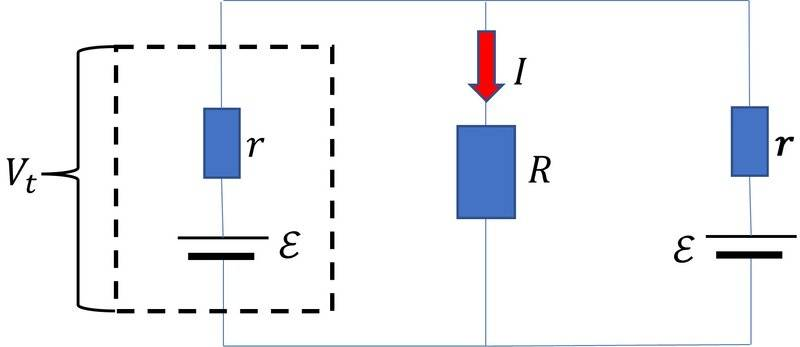I think the relation would be?

$$V_t = \mathcal{E} - \frac{I}{2} r$$

Last edited:
nasu said:
The drop in the load voltage is not a fluctuation. It is normal behavior of the battery. The voltage decreases with time and the decrease rate is faster for larger currents. This is what you see during some battery tests based on cyclic charge and discharge. If you want a practically constant voltage over the duration of your test you need to draw an appropriate low current.

This is a really good point. I encountered this when working with lead-acid batteries for a suborbital experiment; I am not sure if it applies to alkaline cells. But for lead-acids, the battery capacity rating (in A*h) from the manufacturer only applies at one specific assumed/nominal discharge current. If you discharge the battery at higher currents, the corresponding discharge time will reduce faster than linearly in the current. So your battery capacity is discharge-current-dependent, rather than being a constant. There is an empirical relation for this called Peukert's Law.

I observed this behaviour for alkaline and carbon zinc batteries. And for NiMH rechargeables as well. I suppose the concept of "internal resistance" is an useful model but it is not like the resistance of a resistor. You change the model to fit the experimental conditions which may actually mean changes in the diffusion layers around electrodes.

What am I doing wrong?Using the principal of superposition, one of the sources is replaced with a short circuit equivalent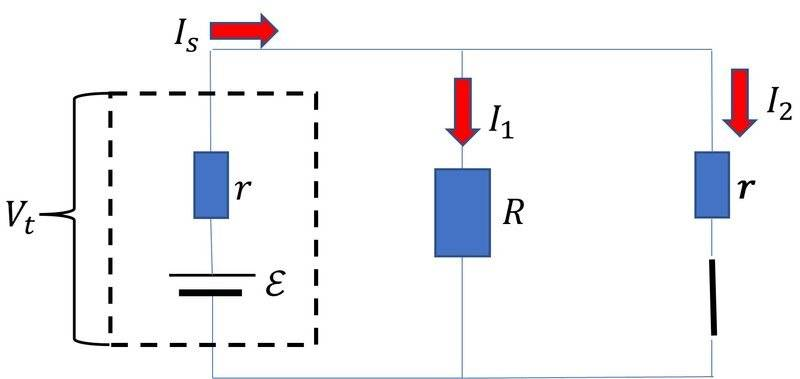After combining ##R## and ##r## and applying Kirchoff's Voltage Law:

$$\mathcal{E} - I_s r - I_s \frac{Rr}{R+r} = 0 \tag{1}$$

Which implies:

$$I_s = \frac{R+r}{r^2+2Rr}\mathcal{E} \tag{2}$$

To determine the current passing through the load ##R## from source 1:

$$I_s = I_1 + I_2 \tag{3}$$

We also have that:

$$I_1 R = I_2 r \implies I_2 = I_1 \frac{R}{r} \tag{4}$$

Substituting ##(4) \to (3)##:

$$I_1 = \frac{r}{R+r} I_s \tag{5}$$

Substitute ##(5) \to (2)##:

$$I_1 = \frac{R+r}{r^2+2Rr} \mathcal{E} \frac{r}{R+r} \tag{6}$$

We repeat the process for the other source and add the results. In this case we find that what we measure as the load current ##I = 2 I_1##

$$I = \frac{2r}{r^2 + 2 R r} \mathcal{E} \tag{7}$$

So to get the value for ##r##, we have ##\mathcal{E}, I, R## and solve ##(7)##:

$$I r^2 + 2 \left( IR - \mathcal{E} \right) r = 0$$

$$\implies r = 2 \left( \frac{\mathcal{E}}{I} - R \right)$$

Last edited:
neilparker62 said:
I am trying to do that. Per setup in my photo above, when I'm ready to take readings I hold the black probe in place on the negative terminal and then touch/release the positive probe holding it just long enough to take a reading. That's why I need to try and stabilize load voltage as best I can.
The number of seconds it is connected to the load is more significant than the number of seconds to do the measurements. Make sure the load is not connected only while measuring.

•.Scott and neilparker62
nasu said:
The voltage under load for a given load decreases as well. I am not talking about the drop (from the open circuit value) you see when you connect the load. The value you measure after connecting the load changes in time. Even the open circuit value right after disconnecting the load may be less than the original emf. It takes a while to get back to the original value.
Yes - this was exactly our experience when attempting to measure load voltage. That's why I attempted to rather use the IR product for load voltage. But then ran into the difficulty that IR was significantly less than (any of) the changing load voltage measurements. Still trying to figure out why but if there's a capacitor involved in a better circuit model, perhaps we're dealing with a long RC time constant ?

Apologies if I misunderstood you on the voltage drop.

anorlunda said:
The number of seconds it is connected to the load is more significant than the number of seconds to do the measurements. Make sure the load is not connected only while measuring.
The lead to the load is connected to the multimeter probe so only gets connected during "touch/release" voltage measurement.

The pro way to do this in a complete EE lab is to do an AC ohm measurement. You can buy instruments designed for this, but you can also set up your own.

The idea here is to use a load (typically a current source) that can be modulated with an AC signal, let's say at 100Hz. Then you measure the voltage and current with a narrow band AC voltmeter that rejects all non-carrier frequencies. This will eliminate all signals that aren't at the modulated frequency. This will give you good measurements for the impedance at the modulated frequency. It is reasonable to assume that the 100Hz impedance is the same as the DC resistance. Of course if you chose 1MHz, you couldn't extrapolate that far.

In a physics lab, a lock-in amplifier would be perfect for these measurements. We did this to measure the resistance of TECs which are a sign of incipient failures. Which is essentially the same problem.

This reminds me of an undergrad EE lab where our assignment was to measure the open loop gain of a uA741, without using feedback! It was barely possible but an incredible PIA. The underlying lesson was, if your measurements are really hard or aren't giving good data, stop and think, or research, a better way.

•neilparker62
erobz said:
What am I doing wrong?

View attachment 303902

Using the principal of superposition, one of the sources is replaced with a short circuit equivalent

View attachment 303901

After combining ##R## and ##r## and applying Kirchoff's Voltage Law:

$$\mathcal{E} - I_s r - I_s \frac{Rr}{R+r} = 0 \tag{1}$$

Which implies:

$$I_s = \frac{R+r}{r^2+2Rr}\mathcal{E} \tag{2}$$

To determine the current passing through the load ##R## from source 1:

$$I_s = I_1 + I_2 \tag{3}$$

We also have that:

$$I_1 R = I_2 r \implies I_2 = I_1 \frac{R}{r} \tag{4}$$

Substituting ##(4) \to (3)##:

$$I_1 = \frac{r}{R+r} I_s \tag{5}$$

Substitute ##(5) \to (2)##:

$$I_1 = \frac{R+r}{r^2+2Rr} \mathcal{E} \frac{r}{R+r} \tag{6}$$

We repeat the process for the other source and add the results. In this case we find that what we measure as the load current ##I = 2 I_1##

$$I = \frac{2r}{r^2 + 2 R r} \mathcal{E}$$
My thinking is that two batteries in parallel have an equivalent circuit (assuming the simple resistive cell model) of a single emf and half the resistance - also assuming both have the same internal resistance.

•erobz
neilparker62 said:
My thinking is that two batteries in parallel have an equivalent circuit (assuming the simple resistive cell model) of a single emf and half the resistance - also assuming both have the same internal resistance.
So how much do the values differ for ##r##? I ask, because I didn't just make it up. That is a standard method to analyze multi source circuits. I obtained it from my textbook. There must be a reason for the theory, and I wonder if it will show up experimentally?

Using:

$$V_t = \mathcal{E} - \frac{1}{2}Ir$$

I get:

$$r = 2 \left( \frac{\mathcal{E}}{I} - R \right) = 2 \left( \frac{V_t + \frac{1}{2}Ir }{I} - R \right) \implies R = \frac{V_t}{I}$$

Which makes perfect sense?

EDIT:
Yeah, they are one and the same, I didn't catch that. If you rearrange ## r = 2 \left( \frac{\mathcal{E}}{I} - R \right) ## with the substitution that ##V_t = I R## you get right back to the first equation...Sorry for the detour.

Last edited:
DaveE said:
The pro way to do this in a complete EE lab is to do an AC ohm measurement. You can buy instruments designed for this, but you can also set up your own.
Or use a pulse test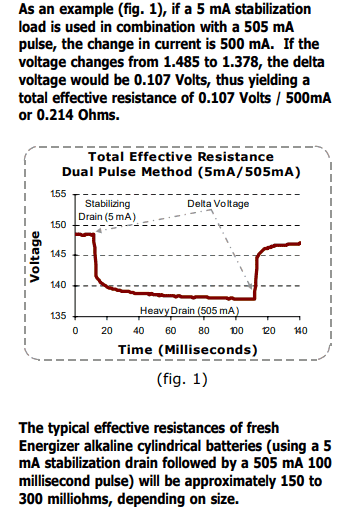•DaveE
neilparker62 said:
Still trying to figure out why but if there's a capacitor involved in a better circuit model, perhaps we're dealing with a long RC time constant ?
At the start of a high current test, you are dealing with the immediate availability of ions at and between the electrode surfaces.

Later in the test, you are dealing with the ion diffusion rate that is needed to keep the test current flowing. That will be temperature dependent.
https://en.wikipedia.org/wiki/Arrhenius_equation

After the test, you are dealing with the time needed for the recovery of chemical equilibrium. That is dependent on the temperature and the amount of charge drawn in earlier tests.

•neilparker62
neilparker62 said:
Or use a pulse test

View attachment 303911
Yes this is also better than a DC measurement. But there is the complexity of either making a really square pulse or dividing two time domain waveforms. Narrow band voltmeters (like in a frequency response analyzer) are easier to get good data out of. Even if your modulation is a bit wonky (harmonics, etc.) the filters will only measure the fundamental. While the impedance at, let's say, 100Hz may not be exactly the whole story (see @Baluncore, above), it is well defined and relatively easy to measure accurately. In addition you can add in the modulation without perturbing the circuit parameters (bias points, etc.). So with an FRA you could characterize impedance vs. load current, or vs. frequency, for example.

•neilparker62
Your graph suggests you are getting errors less than 1%, which is better than any electronic meter I've used. So are you confident that the errors are not in the meter?